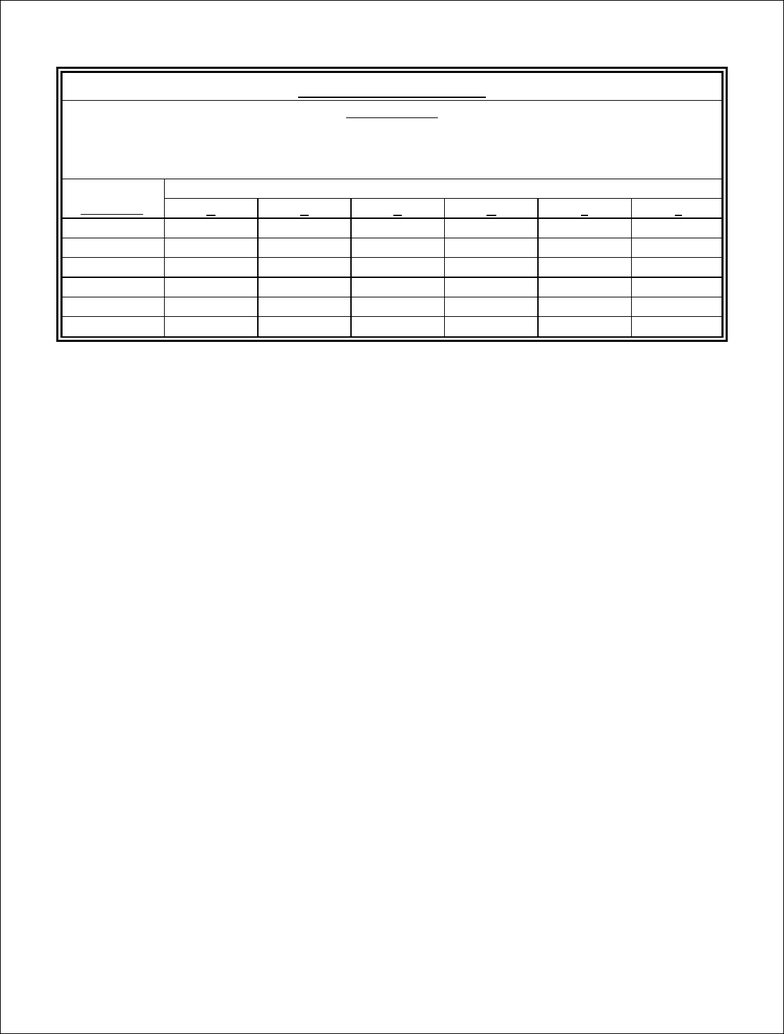Study Guides (380,000)
CA (150,000)
UOttawa (10,000)
ECO (300)
Midterm

# ECO1192 Midterm: Practice Exam #1(1).pdf

Department
Economics
Course Code
ECO 1192
Professor
Claude Théorêt
Study Guide
Midterm

This preview shows pages 1-3. to view the full 9 pages of the document.Page 1
1. The baseline for the acceptance or rejection of a project using the Present Worth
Method is
a) MARR
b) Recovery period less than the industry threshold
c) \$0
d) 1
e) None of the above answers.
2. The baseline for the acceptance or rejection of a project using the Internal Rate of
Return Method (IRR) is
a) MARR
b) Recovery period less than the industry threshold
c) \$0
d) 1
e) None of the above answers.
3. If the rate of interest is 12% compounded quarterly, the effective (annual) rate of
interest is
a) 3%
b) 12%
c) 12.55%
d) None of the above answers.
Engineering Economics
ECO 1192
Practice Examination #1

Only pages 1-3 are available for preview. Some parts have been intentionally blurred.Page 2
4. A common period of analysis must be used with the Present Worth Method to
determine the economic validity of two projects with unequal lives.
a) True
b) False
5. A common period of analysis must be used with the Annual Equivalent Worth or
Annuity Method (AEW) to determine the better of two projects with unequal lives.
a) True
b) False
6. If the rate of interest is 12% compounded monthly, the actual (monthly) rate of interest
is
a) 12%
b) 12.68%
c) 1%
d) None of the above answers.
7. A project with a negative annual equivalent worth (AEW) must have a negative internal
rate of return.
a) True
b) False
8. A project with a Net Present Worth (NPW) = \$0 must have a rate of return
a) Less than MARR
b) Greater than MARR
c) Equal to MARR.
9. A nominal rate of interest will exceed its corresponding effective rate of interest when
a) simple interest (as opposed to compound interest) is used.
b) a project has an infinite life.
c) the effective rate of interest exceeds MARR.
d) None of the above answers.
10. Which nominal (annual) rate of interest compounded semi-annually is equivalent to a
monthly rate of 1%?
a) 12%
b) 6%
c) 6.152%

Only pages 1-3 are available for preview. Some parts have been intentionally blurred.Page 3
QUESTIONS 11 to 14
Projects A to F
are ranked in ascending order of their first cost
have identical lives (N)
have negligible salvage values (SV=0).
PROJECTS
RATES OF RETURN
A
B
C
D
E
F
A
26
-
-
-
-
-
B
24
25
-
-
-
-
C 22 24 22 - - -
D 20 21 20 20 - -
E
19
18
19
18
18
-
F
15
16
17
16
16
17
11. With MARR = 15% and no capital rationing, valid independent projects are:
a) A, B, C, D and E
b) A, B, C, D, E and F
c) E
e) None of the above answers.
12. With MARR = 23% and no capital rationing, valid independent projects are:
a) A and B
b) A, B and C
c) A
d) None of the above answers.
13. With MARR = 15% and no capital rationing, the best mutually exclusive project is:
a) A
b) C
c) D
d) E
e) None of the above answers.
14. With a MARR = 23% and no capital rationing, the best mutually exclusive project is:
a) A
b) B
c) D
d) E
e) F
15. The Internal Rate of Return (IRR) method assumes that cash inflows generated by a
project will be reinvested at the MARR.